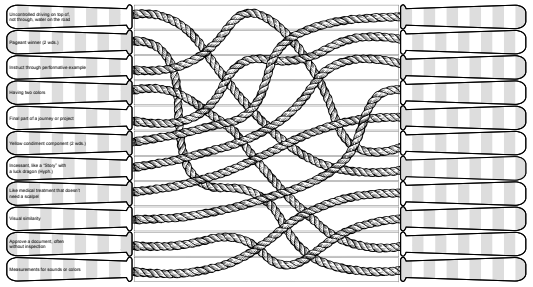## Knot a Puzzle Solution

### by Ata Gurpinar

The rope segments can be rearranged like a jigsaw puzzle and placed in the positions on the jumprope to form the following diagram:As hinted by the flavor text mentioning "eleven-year-olds" and the eleven segments on each jump rope handle, the answer to each clue is eleven letters long, and they have been provided in alphabetical order by answer:

• Uncontrolled driving on top of, not through, water on the road. - AQUAPLANING
• Pageant winner (2 wds.) - BEAUTY QUEEN
• Instruct through performative example - DEMONSTRATE
• Having two colors - DICHROMATIC
• Final part of a journey or project - HOMESTRETCH
• Yellow condiment component - MUSTARD SEED
• Incessant, like a "story" with a luck dragon (Hyph.) - NEVER-ENDING
• Like a medical treatment that doesn't need a scalpel - NONSURGICAL
• Visual similarity - RESEMBLANCE
• Approve a document, often without inspection - RUBBERSTAMP
• Measurements for sound or colors - WAVELENGTH

As the ropes indicate, copying the entries to the connected to the handles on the right, results in the following arrangement. Since there are a number of entries equal to the number of letters in each entry, reading down the diagonal spells an instruction:

 M U S T A R D S E E D H O M E S T R E T C H N E V E R E N D I N G R E S E M B L A N C E N O N S U R G I C A L D E M O N S T R A T E A Q U A P L A N I N G W A V E L E N G T H S R U B B E R S T A M P D I C H R O M A T I C B E A U T Y Q U U E N

The message MOVE US AGAIN instructs the solver to perform the same rearrangement of answers a second time. If needed, the answers can be rewritten on the left handles in this new order and then copied to the connected handles on the right:

 D E M O N S T R A T E N O N S U R G I C A L A Q U A P L A N I N G R U B B E R S T A M P W A V E L E N G T H S N E V E R E N D I N G M U S T A R D S E E D B E A U T Y Q U E E N D I C H R O M A T I C R E S E M B L A N C E H O M E S T R E T C H

Again reading down the diagonal provides the solution DOUBLE DUTCH.